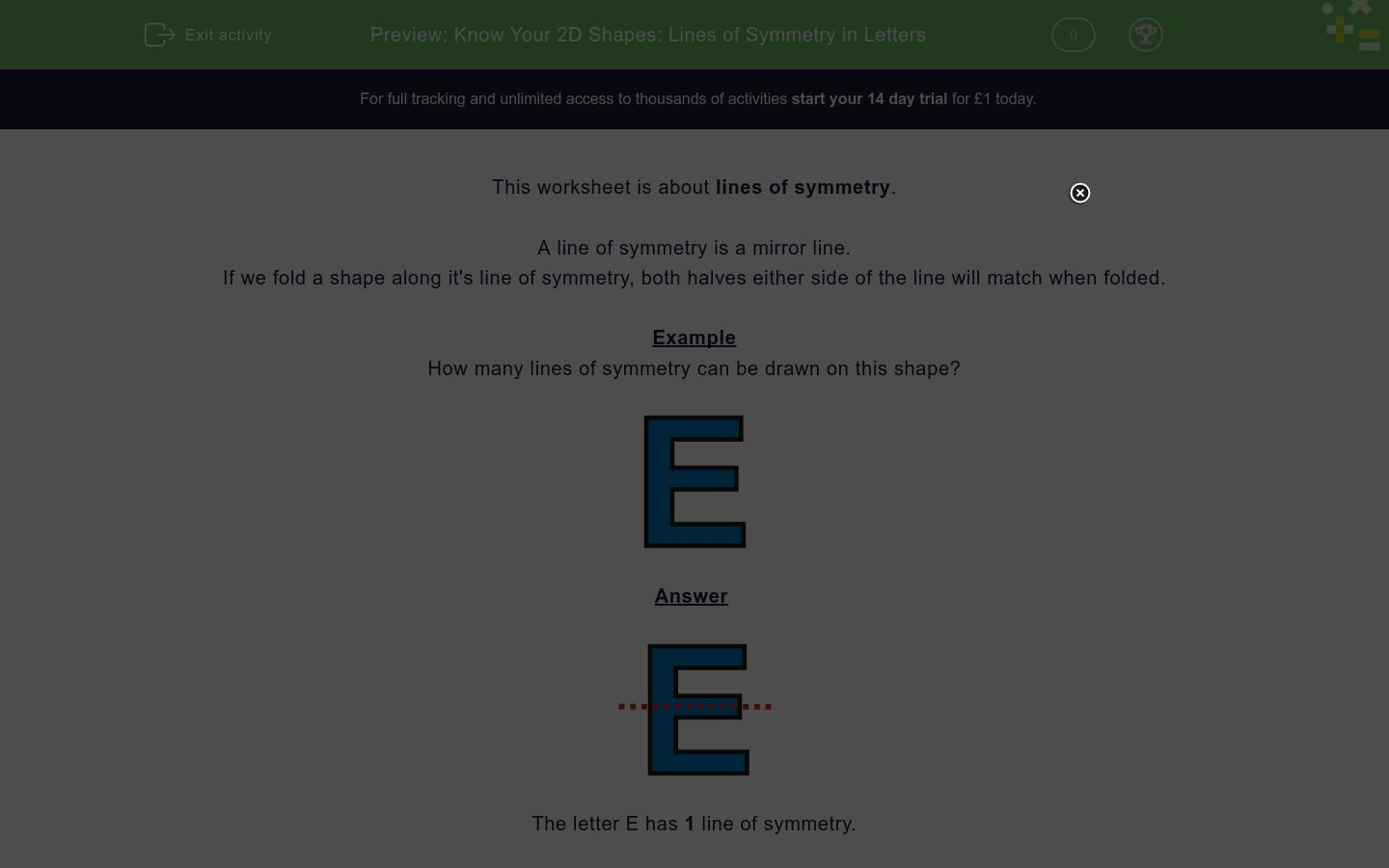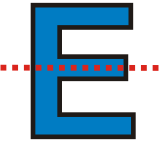# Know Your 2D Shapes: Lines of Symmetry in Letters

In this worksheet, students state how many lines of symmetry a letter has.Key stage:  KS 2

Curriculum topic:   Geometry: Properties of Shapes

Curriculum subtopic:   Identify Line Symmetry in 2D Shapes

Difficulty level:### QUESTION 1 of 10

This worksheet is about lines of symmetry.

A line of symmetry is a mirror line.

If we fold a shape along it's line of symmetry, both halves either side of the line will match when folded.

Example

How many lines of symmetry can be drawn on this shape?The letter E has 1 line of symmetry.

Want to understand this further and learn how this links to other topics in maths?
Why not watch this video?

How many lines of symmetry can be drawn on this shape?3

2

1

How many lines of symmetry can be drawn on this shape?3

2

1

How many lines of symmetry can be drawn on this shape?3

2

1

How many lines of symmetry can be drawn on this shape?1

2

3

How many lines of symmetry can be drawn on this shape?2

1

3

How many lines of symmetry can be drawn on this shape?3

2

1

How many lines of symmetry can be drawn on this shape?3

2

1

How many lines of symmetry can be drawn on this shape?3

2

1

How many lines of symmetry can be drawn on this shape?3

2

1

How many lines of symmetry can be drawn on this shape?3

2

1

• Question 1

How many lines of symmetry can be drawn on this shape?1
EDDIE SAYS
The letter Y has one line of symmetry down the middle vertically.
• Question 2

How many lines of symmetry can be drawn on this shape?1
EDDIE SAYS
The letter E has one line of symmetry down the middle vertically.
• Question 3

How many lines of symmetry can be drawn on this shape?2
EDDIE SAYS
The letter H has 2 lines of symmetry, one down the middle vertically and one across the middle horizontally.
• Question 4

How many lines of symmetry can be drawn on this shape?1
EDDIE SAYS
The letter A has one line of symmetry down the middle vertically.
• Question 5

How many lines of symmetry can be drawn on this shape?2
EDDIE SAYS
The letter I has two lines of symmetry, one down the middle vertically and one across the middle horizontally.
• Question 6

How many lines of symmetry can be drawn on this shape?1
EDDIE SAYS
The letter M has one line of symmetry down the middle vertically.
• Question 7

How many lines of symmetry can be drawn on this shape?1
EDDIE SAYS
The letter U has one line of symmetry down the middle vertically.
• Question 8

How many lines of symmetry can be drawn on this shape?1
EDDIE SAYS
The letter V has one line of symmetry down the middle vertically.
• Question 9

How many lines of symmetry can be drawn on this shape?1
EDDIE SAYS
The letter W has one line of symmetry down the middle vertically.
• Question 10

How many lines of symmetry can be drawn on this shape?2
EDDIE SAYS
The letter X has two lines of symmetry, one down the middle vertically and one across the middle horizontally.
---- OR ----